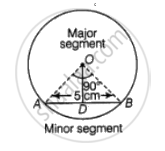# Find the difference of the areas of two segments of a circle formed by a chord of length 5 cm subtending an angle of 90° at the centre. - Mathematics

Sum

Find the difference of the areas of two segments of a circle formed by a chord of length 5 cm subtending an angle of 90° at the centre.

#### Solution

Let the radius of the circle be r.

OA = OB = r cm

We have,

AB = 5 cmCentral angle of AOBA = 90°

In ΔAOB, AB2 = OA2 + OB2

52 = r2 + r2

r = 5/sqrt(2) cm

Also, AD = DB = 5/2 cm

= (5/sqrt(2))^2 - (5/2)^2

= 5/2 cm

Area of isosceles ΔAOB = 1/2 × base × height

= 1/2 x 5 xx 5/2

= 25/4 cm2

Area of sector AOBA = (pir^2)/360 xx theta

= (pi(5/sqrt(2))^2)/360 xx 90

= (25pi)/8

Area of minor segment = Area of sector AOBA - Area of isosceles ΔAOB

= ((25pi)/8 - 25/4)

Now, Area of circle = pir^2

= pi(5/sqrt(2))^2

= (25pi)/2

Area of major segment = Area of circle  – Area of minor segment

= (25pi)/2 - ((25pi)/8 - 25/4)

= ((75pi)/8 + 25/4)

Now, Difference = Area of major segment Δ Area of minor segment

= ((75pi)/8 + 25/4) - ((25pi)/8 - 25/4)

= (25pi)/4 + 25/2  cm^2

Concept: Areas of Sector and Segment of a Circle
Is there an error in this question or solution?

#### APPEARS IN

NCERT Mathematics Exemplar Class 10
Chapter 11 Area Related To Circles
Exercise 11.4 | Q 19 | Page 135
Share